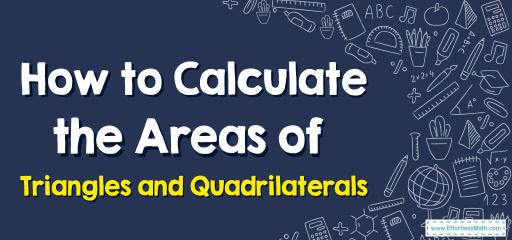# How to Calculate the Areas of Triangles and Quadrilaterals

Triangles and quadrilaterals are two of the most fundamental shapes in geometry, forming the building blocks for more complex shapes and structures. Understanding how to calculate their areas is a vital skill in various fields, from architecture to art. This blog post seeks to provide a comprehensive guide to the areas of triangles and quadrilaterals, taking you through a journey of understanding, one step at a time.## Step-by-step Guide: Areas of Triangles and Quadrilaterals

1. Triangles: A triangle is a three-sided polygon.
Area: The area $$A$$ of a triangle with base $$b$$ and height $$h$$ perpendicular to the base is:
$$A = \frac{1}{2} b \times h$$

Area of a Rectangle: For a rectangle with length $$l$$ and width $$w$$, the area $$A$$ is:
$$A = l \times w$$
Area of a Square: For a square with side $$s$$, the area $$A$$ is:
$$A = s^2$$
Area of a Trapezoid: For a trapezoid with parallel sides (bases) $$a$$ and $$b$$, and height $$h$$ perpendicular to the bases, the area $$A$$ is:
$$A = \frac{1}{2} (a + b) \times h$$
Area of a Parallelogram: For a parallelogram with base $$b$$ and height $$h$$ perpendicular to the base, the area $$A$$ is:
$$A = b \times h$$
Area of a Rhombus: The area $$A$$ of a rhombus with diagonals $$d_1$$ and $$d_2$$ is:
$$A = \frac{1}{2} d_1 \times d_2$$

### Examples

Example 1:
Calculate the area of a trapezoid with bases $$7 \text{cm}$$ and $$5 \text{cm}$$, and a height of $$6 \text{cm}$$.

Solution:
$$A = \frac{1}{2} (7 + 5) \times 6 = 36 \text{cm}^2$$.

Example 2:
If a rhombus has diagonals measuring $$10 \text{cm}$$ and $$8 \text{cm}$$, what’s its area?

Solution:
$$A = \frac{1}{2} \times 10 \times 8 = 40 \text{cm}^2$$.

Example 3:
Calculate the area of a triangle with a base of $$10 \text{cm}$$ and a height of $$5 \text{cm}$$.

Solution:
$$A = \frac{1}{2} \times 10 \times 5 = 25 \text{cm}^2$$.

Example 4:
If the area of a triangle is $$30 \text{cm}^2$$ and its base is $$10 \text{cm}$$, find its height.

Solution:
Using the formula:
$$30 = \frac{1}{2} \times 10 \times h$$
Solving for (h), we get:
$$h = 6 \text{cm}$$

### Practice Questions:

1. Determine the area of a triangle with a base of $$12 \text{cm}$$ and a height of $$8 \text{cm}$$.
2. Calculate the area of a square with a side length of $$6 \text{cm}$$.
3. What is the area of a parallelogram with a base of $$9 \text{cm}$$ and a height of $$5 \text{cm}$$?
4. A rhombus has one diagonal measuring $$15 \text{cm}$$. If its area is $$45 \text{cm}^2$$, find the length of the other diagonal.

1. $$48 \text{cm}^2$$.
2. $$36 \text{cm}^2$$.
3. $$45 \text{cm}^2$$.
4. $$6 \text{cm}$$.

### What people say about "How to Calculate the Areas of Triangles and Quadrilaterals - Effortless Math: We Help Students Learn to LOVE Mathematics"?

No one replied yet.

X
30% OFF

Limited time only!

Save Over 30%

SAVE $5 It was$16.99 now it is \$11.99